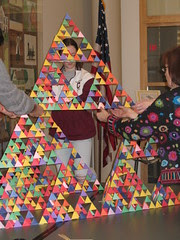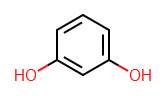Tuesday, 30 November 2010

Conference attendance at what price?

How much does it cost to attend a conference, all in? If your boss is paying then you mightn't care. However, you need to have a figure in mind when you start writing grants because you need to budget it in. Want to go to one European and one international conference a year for three years? How much would that set you back?

I recently attended the German Conference on Cheminformatics in Goslar, and I determined that this time, at least, I would do my utmost (short of squabbling over the bill) to collect all relevant receipts as I had specific funding just for those costs.

Now, bearing in mind that Cork->Goslar is an indirect flight involving an overnight stay plus some train journeys here are the figures (rounded to the nearest 20 euro, E&OE):

Registration: 280
Poster: 60
Accommodation: 220
Trains: 100
Taxis: 60
Flights: 180
Food: 120

So, in or around 1000 euro. It does add up, doesn't it? Going to the ACS I would guesstimate at around 2.5 times this. Anyone got a better estimate?

Thursday, 25 November 2010

Picture this - programming for funAs I said only last year, when I started programming I used to play around with graphics. So here are a couple of programming problems based around graphics, and specifically around the Sierpinski triangle.

Random motion within a triangle

What do you get if you put the following recipe together?

(1) Draw an equilateral triangle and label the corners A, B and C
(2) Set your current position, P, to corner A.
(3) Choose one of the three corners at random and move P halfway to that corner.
(4) Draw a dot at the new location of P.
(5) Go back to 3 (Repeat 500 or 1000 times in total)

Apparently, this is called the Chaos game. If you follow the links on the Wikipedia page I linked to, it seems that the same method could be used to draw a fern fractal.

Triangles within triangles

This one is a bit tricky in terms of programming as you need to learn about recursion (functions that call themselves). It's not rocket science but it's a bit confusing first time you see it. Not to worry - let's just plough ahead anyway :-)

(0) Draw a white equilateral triangle of length M (some value) pointing upwards centered around a point N (some point in the middle of the screen).
(1) Write a function that takes a point on the screen, P, and a length, L, and draws an upside-down black equilateral triangle centered around P with sides of length L. For example, if this function is called with L=M/2 and with P=N, this should leave three white equilateral triangles on the screen (separated by the black one).
(2) The second thing the function should do is if L > M/16, it should call itself three separate times to draw three black triangles of length L/2 centred in the middle of each of the three white equilateral triangles around it.
(3) Now, back in the main part of the program, call the function you've just written using N for the value of P, and M/2 for the value of L.

If you get this working, this is a fun program to play around with. What happens if you use squares or circles? Another related structure is the Koch snowflake: there's a recipe at Wikipedia.

Pascal's Triangle

Pascal's Triangle is a triangle of numbers that looks like this:

1
1 1
1 2 1
1 3 3 1
1 4 6 4 1
1 5 10 10 5 1

Each new row is created by adding the two numbers in the row above it.

To turn this into a nice graphic, create the first 1000 or so rows of the triangle, and draw it on the screen by using a white dot for the odd numbers, and a black dot for the evens.

Image credit: chelmsfordpubliclibrary

Friday, 19 November 2010

Relaxed PES scan using Firefly

I'm interested in analysing the barrier to rotation around a particular bond in a molecule. A simple way to look at this is to fix the torsion angle around the bond, and optimise the other coordinates. The end result is a plot of the energy versus the torsion angle.Here I'll look at the energy profile when rotating around the C-C bond in ethane. There seem to be two approaches to do this using Firefly. The first is described at the Firefly website and involves using IFREEZ in the STATPT group.

However, this approach seems to require users to spell out the internal coordinates themselves. Instead, it's easier to have Firefly work out the coordinates itself. After some hacking based on examples in the docs (see links below), I came up with the following which appears to give the same results but I think is more generally useful:
\$CONTRL RUNTYP=rsurface NZVAR=18 SCFTYP=RHF COORD=CART UNITS=BOHR
MAXIT=50 \$END
\$SYSTEM TIMLIM=500 MEMORY=30000000 \$END
\$BASIS GBASIS=n31 ngauss=6 \$END
! To scan along the torsion 6,2,1,4 from 0->120 in steps of 4
\$zmat dlc=1 auto=1 ifzmat(1)=3,6,1,2,4 scan=.t. DLCTOL=1D-7 ORTTOL=1D-7
ifdmod=2 \$end
\$surf ndisp1=31 disp1=4 orig1=0 reuse=.t. \$end
\$DATA
CH3-CH3
C1
C 6.0 0.0000000000 0.0000000000 -1.4550890105
C 6.0 0.0000000000 0.0000000000 1.4550890105
H 1.0 1.9475804164 0.0000000000 -2.1256947270
H 1.0 -0.9737902082 -1.6866541165 -2.1256947270
H 1.0 -0.9737902082 1.6866541165 -2.1256947270
H 1.0 -0.9737902082 -1.6866541165 2.1256947270
H 1.0 1.9475804164 0.0000000000 2.1256947270
H 1.0 -0.9737902082 1.6866541165 2.1256947270
\$END
Note: the IFDMOD=2 was added as suggested on the Firefly mailing list, as I originally got the error message "UNABLE TO PROJECT DLC!".

Docs: [Overview] [Full list of options]

Notes to self:
(1) Firefly was run on Windows as follows:
set PATH=C:\Tools\firefly;%PATH%
set INPUT=myinputfile.txt
firefly -f > myoutputfile.txt
(2)For the actual system of interest, I found that the initial conversion of the 'displacement' from internal to cartesian coordinates failed to converge until I set the starting angle for the scan to close to the angle in the input data.
(3) The graph was created using the following Python code:

Wednesday, 17 November 2010

Name that graph - the reveal

Here's a graph I created showing the relationship between the first few elements of the periodic table and...what?Every year around November the ACS sends me a mug corresponding to a different chemical element. The first year ("H") the mug arrived in one piece...:-)

Tuesday, 16 November 2010

Presenting Confab at Goslar, and the Jacques-Émile Dubois Grant

Last week I attended the 6th German Conference on Chemoinformatics at Goslar for the first time. This was thanks to the Jacques-Émile Dubois Grant from the CSA Trust which covered the cost of my attendance. There aren't many awards available for young cheminformaticians, so if you're 35 or younger think about applying (it's open right now) - it's a short and simple application, and could cover the cost of attending a conference or visiting another group.

Here's the poster I presented on Confab:

View more documents from baoilleach.

Name that graph

Here's a graph I created showing the relationship between the first few elements of the periodic table and...what?Don't take it too seriously, as it's not quite a scientific study, but here's a hint: every year around November I will be able to add the next element to the graph.

Update (17/11/2010): The answer

Thursday, 4 November 2010

Automorphisms, isomorphisms, symmetry classes and canonical labellingI've just been spending some time ensuring that Tim's new symmetry and isomorphism code in Open Babel can be accessed from Python (you can already access it from C++). It didn't make it into the 2.3.0 official release but I'll push it out into the Windows Python in the next while.

First the symmetry classes and canonical labelling. These can be calculated and accessed as follows (the test molecule is shown in the image above):
import pybel
ob = pybel.ob

mol = pybel.readstring("smi", "c1c(O)cccc1(O)")

gs = ob.OBGraphSym(mol.OBMol)
symclasses = ob.vectorUnsignedInt()
gs.GetSymmetry(symclasses)

print symclasses
# <openbabel.vectorUnsignedInt; proxy of <Swig Object of type 'std::vector< unsigned int > *' at 0x004DFE90> >
print list(symclasses)

# [4, 5, 1, 3, 2, 3, 5, 1]

canonlabels = ob.vectorUnsignedInt()
ob.CanonicalLabels(mol.OBMol, symclasses, canonlabels)
print list(canonlabels)

# [4, 2, 1, 3, 5, 7, 6, 8]

Does the molecule have any automorphisms?
automorphs = ob.vvpairUIntUInt()
ob.FindAutomorphisms(mol.OBMol, automorphs, symclasses)
for x in automorphs:
print x

# ((0, 0), (1, 6), (6, 1), (2, 7), (7, 2), (3, 5), (5, 3), (4, 4))
# ((0, 0), (1, 1), (2, 2), (3, 3), (4, 4), (5, 5), (6, 6), (7, 7))

Next we'll use the example molecule as a query to see how it matches another identical molecule which has the atoms in a different order:
query = ob.CompileMoleculeQuery(mol.OBMol)
mapper = ob.OBIsomorphismMapper.GetInstance(query)

mol_b = pybel.readstring("smi", "c1(O)cccc(O)c1")

isomorph = ob.vpairUIntUInt()
mapper.MapFirst(mol_b.OBMol, isomorph)
print list(isomorph)

# [(0, 7), (1, 5), (3, 4), (4, 3), (5, 2), (6, 0), (2, 6), (7, 1)]

isomorphs = ob.vvpairUIntUInt()
mapper.MapAll(mol_b.OBMol, isomorphs)
for x in isomorphs:
print x

# ((0, 7), (1, 5), (3, 4), (4, 3), (5, 2), (6, 0), (2, 6), (7, 1))
# ((0, 7), (1, 0), (2, 1), (3, 2), (4, 3), (5, 4), (6, 5), (7, 6))